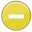#kilometer 中文解釋 wordnet sense Collocation Usage Collins Definition
Noun
/kiˈlämitər/,/ˈkiləˌmētər/,Font size:kilometers, plural; kilometres, plural;
1. A metric unit of measurement equal to 1,000 meters (approximately 0.62 miles)

1. a metric unit of length equal to 1000 meters (or 0.621371 miles)
2. The kilometre (American spelling: kilometer), symbol km is a unit of length in the metric system, equal to one thousand metres and is therefore exactly equal to the distance travelled by light in free space in of a second. ...
3. US spelling of kilometre
4. (kilometers) clicks (slang) Canada  (and Australia?)
5. is a unit for measuring length. The average length between Earth’s center and its surface is about 6,000 Kilometers.
6. is a metric unit of distance equal to 3,280.8 feet or 0.621 miles. Index
7. 1000 meters. A kilometer equals 0.6214 miles.
8. Measurement of distance they tried to convert you to in elemetray school. Equal to .62 miles. MFG courses are measured in kilometers and will range form 2.5 – 3.0 KM. Long enough for great racing, short enough for great spectating.
9. A unit of distance corresponding to 1000m.
10. (km) A unit of length of 1000 meters. 1 km = 0.6213712 miles (about 5/8 mile); 1 mile = 1.609344 km. In Canada it is pronounced KILL-oh-mee-ter, but elsewhere it is pronounced kull-LAW-mitt-er. Colloquially it is referred to as a klik or klick
11. A unit of measurement used on charts to further confuse pilots who already have trouble with knots.
12. A metric measurement commonly used in athletic distances. Abbreviated Km or K. 1K equals 0.62 miles. Inversely, 1 mile equals 1.62 kilometers. 5K is 3.1 miles, while 10K (this is higher math) is 6.2 miles.
13. 1,000 meters or total running distance required to burn half a donut!
14. One thousand meters or 3,281 feet. The kilometer is a unit of measurement for fiber optics.
15. a measure of distance used in almost all other countries, at about 5/8 mile.